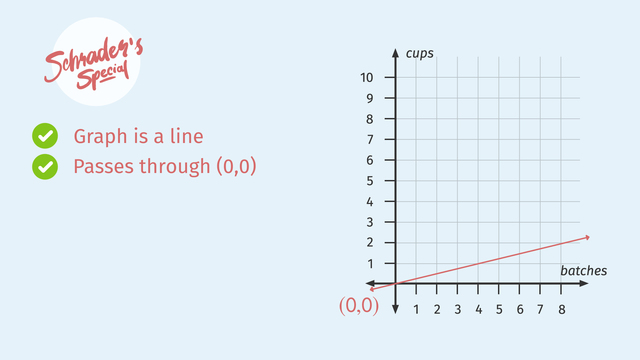# Equations of Graphs of Proportional Relationships Involving FractionsRate this video

Be the first to rate this video!

The authorChris S.

## Information about the videoEquations of Graphs of Proportional Relationships Involving Fractions

After this lesson you will be able to solve proportional relationship problems involving fractions, using tables, graphs, and equations.

The lesson begins with a review of the graphs of proportional relationships and how to writing an equation using the constant of proportionality. This leads to a method for generating a table of values, which can be verified by graphing.

Learn about proportional relationships by helping the scouts win the Cookie Combat Championships!

This video includes key concepts, notation, and vocabulary such as: proportional relationship (relationship between two equal ratios); linear relationship (represented by a line on the coordinate plane, where the slope or rate of change always stays the same); ordered pair (two corresponding values in a proportional relationship); the constant of proportionality (in a proportional relationship, the constant which is equal to the slope of the line); and origin (the point (0,0) on the coordinate plane).

Before watching this video, you should already be familiar with complex fractions; interpreting ratios represented in tables, equations and graphs; converting between those representations; and solving part-to-part and part-to-whole problems using a variety of tools.

After watching this video, you will be prepared to learn more about scale factors and geometric representations of proportionality.

Common Core Standard(s) in focus: 7.RP.A.1, 7.EE.B.4a A video intended for math students in the 7th grades Recommended for students who are 12-13 years old